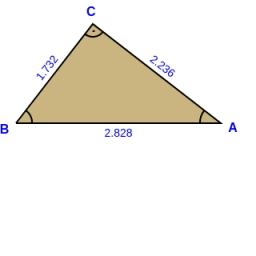# Is right-angled

Can a triangle with the sides of sqrt 3, sqrt 5 and sqrt 8 (√3, √5 and √8) be a right triangle?

y =  1

### Step-by-step explanation:

Try calculation via our triangle calculator.Did you find an error or inaccuracy? Feel free to write us. Thank you!Tips to related online calculators
Pythagorean theorem is the base for the right triangle calculator.

#### You need to know the following knowledge to solve this word math problem:

We encourage you to watch this tutorial video on this math problem:

## Related math problems and questions:

• Is right?Is a triangle with sides 51, 56, and 77 right triangle?
• Quarter circleWhat is the radius of a circle inscribed in the quarter circle with a radius of 100 cm?
• The sides 2The sides of a trapezoid are in the ratio 2:5:8:5. The trapezoid's area is 245. Find the height and the perimeter of the trapezoid.
• GardenThe area of a square garden is 2/9 of triangle garden with sides 160 m, 100 m, and 100 m. How many meters of fencing need to fence a square garden?
• Triangle SASCalculate the triangle area and perimeter, if the two sides are 51 cm and 110 cm long and angle them clamped is 130 °.
• Square 2Points D[10,-8] and B[4,5] are opposed vertices of the square ABCD. Calculate area of the square ABCD.
• Sum of squaresThe sum of squares above the sides of the rectangular triangle is 900 cm2. Calculate content of square over the triangle's hypotenuse.
• Right triangleLegs of the right triangle are in the ratio a:b = 2:8. The hypotenuse has a length of 87 cm. Calculate the perimeter and area of the triangle.
• Right angled triangle 2LMN is a right-angled triangle with vertices at L(1,3), M(3,5), and N(6,n). Given angle LMN is 90° find n
• Right angled triangle 3Side b = 1.5, hypotenuse angle A = 70 degrees, Angle B = 20 degrees. Find its unknown sides length.
• SquarePoints A[9,9] and B[-4,1] are adjacent vertices of the square ABCD. Calculate the area of the square ABCD.
• Sides of right angled triangleOne leg is 1 m shorter than the hypotenuse, and the second leg is 2 m shorter than the hypotenuse. Find the lengths of all sides of the right-angled triangle.
• Triangle ABCRight triangle ABC with right angle at the C, |BC|=18, |AB|=33. Calculate the height of the triangle hAB to the side AB.
• Right isoscelesCalculate area of the isosceles right triangle which perimeter is 26 cm.
• Without Euclid lawsRight triangle ABC with right angle at the C has a=14 and hypotenuse c=26. Calculate the height h of this triangle without the use of Euclidean laws.
• Right angleIf a, b and c are two sides of a triangle ABC, a right angle in A, find the value on each missing side. If b=10, c=6
• ChordIn a circle with radius r=60 cm is chord 4× longer than its distance from the center. What is the length of the chord?